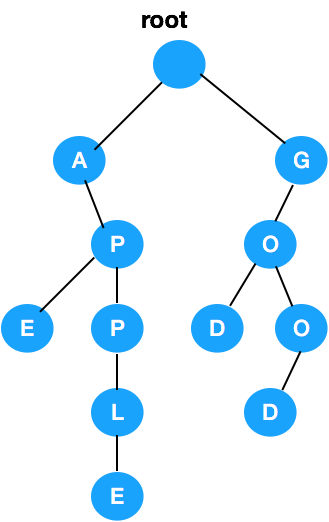# Java and Beyond# Design Search AutoCompletion using plain Java

This article intends to discuss the low-level implementation of Search Autocompletion using Java. Let's cut the crap and directly mention that we will be using `Trie` data structure for the use case. We're not going to discuss in depth about `Trie` data structure or why it is the right fit here. There are plenty of HLD articles around for you to refer to. So, let's focus on the implementation here.

Here is a sample `TrieNode` class:

``````class TrieNode{
Map<Character,TrieNode> children;
boolean isEndOfWord;

TrieNode(){
children = new HashMap<>();
isEndOfWord = false;
}
}
``````

Note that, we're using `Map` here for storing all characters at a particular level in the `Trie`. If the question is to return the results in alphabetic order, then we got no option but to use `TreeMap`. This will ensure the order but will cost you in insertion and search since `TreeMap` lookups/insertions would take `log(n)` time.

For this article, we assume that we can return results in any order to make things simple. Also, let's assume we have the cached response from the backend. We can take it as a source for words for the autocompletion task. Let this input be `words` and let's say we're searching for a word `searchWord` . So, the method definition boils down to the below:

``````public List<String> suggestedWords(String[] words,
String searchWord) {
for (String word: words) {
insert(word); // insert to Trie
}
return search(searchWord); // search in Trie and return results
}
``````

The method `insert(String word)` would insert words to the `Trie`. Below is the sample implementation for the same. Note that, we need to set the flag `isEndOfWord` to `true` for the last `TrieNode`, indicating the last character of the word.

``````private void insert(String word) {
int len = word.length();
TrieNode current = this.root; // member variable pointing to the root of TrieNode
for (int i = 0; i < len; i++) {
TrieNode node = current.children.get(word.charAt(i));
if (node == null) {
node = new TrieNode();
current.children.put(word.charAt(i), node);
}
current = node;
}
current.isEndOfWord = true;
}
``````

`Trie` can be prepopulated and can be set up in an application server instead. So, real-time queries just have to call `search(searchWord)` directly. After inserting a bunch of words, it would look like the below (eg: apple, ape, god, good) :Now comes the major part, which is search auto-completion. To start with, we need to implement `prefix` search. We should be able to search for a `prefix` in the `Trie`. If there is a match found, then we need to search for complete words from that specific `TrieNode`. Real-time applications would have `k` number of results returned rather than all words. It makes sense to have `k` words returned for real-time performance. Here is the implementation for `prefix` search:

``````private TrieNode startsWith(String prefix) {
int len = prefix.length();
TrieNode current = this.root;

for (int i = 0; i < len; i++) {
TrieNode node = current.children.get(prefix.charAt(i));
if (node == null) {
return null;
}
current = node;
}
return current;
}
``````

If there are no `prefix` found in the `Trie`, we return `null`, otherwise we return the `TrieNode` will be the starting point for our main search. Prefix search will only take `O(n)` time in the worst case. Let me re-write the `search` function below with all the defined methods so far:

``````private List<String> search(String searchWord) {
List<String> result = new ArrayList <> ();
TrieNode current = this.root;
int len = searchWord.length();

current = startsWith(searchWord);
if (current != null) {
List<String> list = new ArrayList <> ();
StringBuilder sb = new StringBuilder(searchWord);
// backtrack(list, current, sb, k); yet to implement.
}
return result;
}
``````

Note that, `searchWord` may or may not be a `complete` word. But it doesn't matter and we return all `complete` words for the `searchWord` . I have commented on the above code which is yet to be discussed/implemented. We can use `backtracking` to traverse all following `TrieNodes` from the prefix `TrieNode`, until we find a `complete` word. Refer to the below implementation:

``````private void backtrack(List<String> list,
TrieNode current,
StringBuilder sb,
int k) {
if (list.size() == k) {
return;
}
if (current.isEndOfWord) {
}
for (Map.Entry<Character,TrieNode> entry: current.children.entrySet()) {
sb.append(entry.getKey());
backtrack(list, entry.getValue(), sb);
sb.setLength(sb.length() - 1);
}
}
``````

We stop `backtracking` the `Trie` when `at most` `k` words are collected. Most importantly, we collect the `word` with the help of `isEndOfWord` flag. Use of `StringBuilder` is self-explanatory here. Once `backtracking` is done, the `result` will have all the `complete` words. Now, the same solution can be applied if we need to return a list of `phrases` instead of a list of `single words`. The `word` here is synonymous with a `phrase`, so it doesn't matter.

This is something you can quickly code up in an actual coding interview and the sky is the limit for improvements! Happy coding!